Courses

# Procedure - To determine the density of a solid (which is denser than water), Physics, Science Class 9 Notes | EduRev

## Class 9 : Procedure - To determine the density of a solid (which is denser than water), Physics, Science Class 9 Notes | EduRev

The document Procedure - To determine the density of a solid (which is denser than water), Physics, Science Class 9 Notes | EduRev is a part of the Class 9 Course Science Class 9.
All you need of Class 9 at this link: Class 9

Materials Required:

Procedure:

How to measure the gravitational mass of a solid using a spring balance?

In real lab:

• Take a metallic solid block.

• Tie it with a thin strong thread to hang it on the hook of the spring balance.

• Note the least count of the spring balance.

• Hang the block on the hook of spring balance. It is better to hang the spring balance with the help of an iron stand or clamp stand so that it remains static while noting the mass of the block.

• Carefully observe the gravitational mass of the solid block and note it down. Repeat it thrice and calculate mean of the three readings taken.

To perform the simulator:

• Note the least count of the spring balance.

• Click on any one of the given solids such as stone, steel ball, wooden block, copper lock, and copper block to measure its gravitational mass.

• Once you click on it, the selected solid will shown as hung on the spring balance.

• Mouse over the scale on the spring balance where the red indicator points to get the readings.

Now we need to find the Mass of the solid.

Mass of the solid,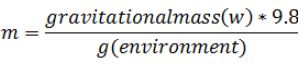Now we need to find the volume of the solid as you know,

Density,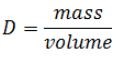a) As performed in a real lab:

• Take a graduated glass cylinder of proper size and capacity. Fill it with water up to a known volume level mark.

• Tie the rectangular metallic block by a thin strong thread and immerse it fully in water taken in the graduated cylinder. The block displaces water and the water level rises. Note the position of water level (meniscus) keeping the eye in horizontal position with the level (to avoid error due to parallax).

• Find the difference of two positions of the water level to find volume of metallic block immersed.

• Repeat it thrice and calculate the mean of three observations taken.

b) As performed using the simulator:

• Click on the button, “Volume” to measure the volume of the solid.

• Click on one of the given solids to measure its volume.

• Now mouse over the cylinder to measure the water rise in the cylinder.

• You can also check your readings on the space given and by clicking on the button, “Check”.

• Calculate the density using the formulae and check your results in the respective space.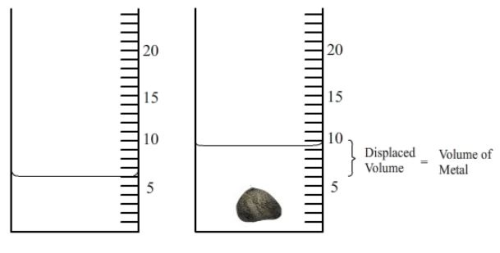Observations:

Record your observation of measurement of mass and volume in the below tables as shown.

1. Table: For gravitational Mass of the Solid (M) Block

 Sl.No Initial reading (without block) M1 Final reading(with solid block) MF Mass of the solid MF-M1 1 M1=............g 2 M2=.............g 3 M3 =............g

Mean gravitational mass of the solid block,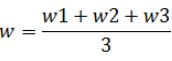=...................g.wt (by spring balance)

2. Table: For Volume of the Solid Block (V)

 Sl.No. Initial reading (without block immersed)V1(ml) Final reading (with block immersed)VF(ml) Volume of the block V=V1-VF(ml) 1 V1=............ml 2 V2=.............ml 3 V3=.............ml

Mean volume of the solid block by graduated cylinder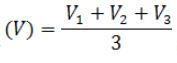= ...................ml(cm3).

When the experiment is conducted in earth as environment, the mass of the object and gravitational mass displayed on the spring balance is the same. But when we do it on another planet like moon, mars, etc., the mass of object and gravitational mass are different. The mass displayed in the spring balance is taken as gravitational mass (w) of the object and the mass of object can be calculated by using the formula.

Mass of the object:

You can calculate the density (D) of the given solid block (denser than water) with the calculated mean mass and volume.

Mass of the solid,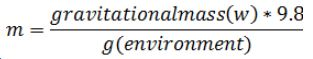(i) Mass of the solid (m) = ..................... g

(ii) Mean volume of the solid block (V) =...................cm3

(iii) Density (D) of the solid block = Mass / Volume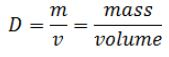=...................... g/cm3

Least count of the spring balance

5 divisions = 25 g

1 division = 25/5 = 5 g

Result:

The density of the given solid (heavier than water) is .................... g/cm3./p>

Precautions:

Let's try the experiment!

1. Always used a thread of least weight and volume to tie the solid block.

2. The solid block should be dried before measuring mass and volume.

3. The indicator of the spring balance should be at zero before measuring the mass of the solid.

4. The solid block should be completely immersed in water of the measuring cylinder before observing its volume.

5. While immersing the solid block, the water of the measuring cylinder should not spill.

6. The solid block should not touch the brim and sides of the beaker.

7. Always note reading of lower meniscus of water level.

Offer running on EduRev: Apply code STAYHOME200 to get INR 200 off on our premium plan EduRev Infinity!

## Science Class 9

143 videos|224 docs|135 tests

,

,

,

,

,

,

,

,

,

,

,

,

,

,

,

,

,

,

,

,

,

,

,

,

,

,

,

;